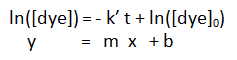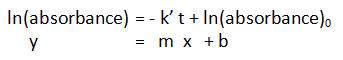# Kinetics Activity

-Introduction

-Background Step 1

-Background Step 2

-Step 1: Making a kinetic trace

-Step 2a: Getting information from a kinetic trace

-Step 2b: Determining the pseudo rate constant k'

-Step 3: Determine the effect of bleach on the reaction

-Step 4: Converting absorbance to concentration

Kinetics Studies of the Bleaching of Food Dyes >> Step 2: Getting information from a kinetic trace

 < Previous | Next >

# Kinetics Studies of the Bleaching of Food Dyes

## Step 2b. Determining the pseudo rate constant k'

For a first order reaction the integrated rate law is:However, we measure the absorbance, which is proportional to the concentration of dye. So we instead write the integrated rate law as:[Note: this takes advantage of the properties of logarithms. The absorbance is proportional to dye concentration (absorbance = const [dye]), and so ln(absorbance) = ln(dye) + ln(const). The ln(const) appears on both the left and right hand side of the integrated rate law, and so cancels: ln(dye) + ln(const) = - k' t + ln(const) + ln(dye)0)]

We plotted ln([absorbance]) on our y axis and time, t, on our x axis. The result is a straight line whose slope is m = - k', and whose intercept is b = ln(absorbance)0. So the slope we measure from our plot gives us the quantity we want, the pseudo rate constant k'.

Determine k' and complete the section of the homework document corresponding to step 2.

At this point, you have determined the order of the reaction with respect to dye, and calculated the pseudo first-order rate constant, k'. What we next need to do is determine the effects of the bleach concentration on the rate of the reaction.

 < Previous | Next >